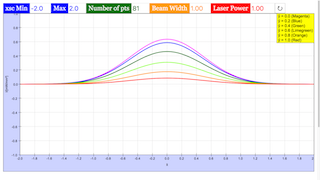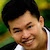## PICUP Laser Beam Profile EXERCISE 1: THE TEM00 MODE: “LINE CUTS” JavaScript Simulation Applet HTML5Intro Page

Laser Beam Profile

Developed by E. Behringer

This set of exercises guides the student to model the results of an experiment to determine the profile of a laser beam using a knife-edge technique. It requires the development of the model of the knife-edge profile, and fitting of the model profile to experimental data. Here, the computational tasks are handled by built-in functions of the computational tool being used to complete these exercises.

 Subject Area Waves & Optics Beyond the First Year Python Students who complete this set of exercises will be able to express an equation predicting the profile of a laser oscillating in the TEM00 mode in terms of dimensionless (“scaled”) variables suitable for coding (Exercise 1); produce both line plots and contour plots of the (scaled) irradiance of the beam versus (scaled) position(s) (Exercises 1 and 2); develop a model of, and plot, the knife-edge profile of the laser beam (Exercise 3); and fit the model, i.e., of the irradiance versus knife-edge position, to experimental data (Exercise 4) 120 min
Exercise 1

### EXERCISE 1: THE TEM00MODE: “LINE CUTS”

The irradiance  (power per unit area) of a laser oscillating in the TEM mode can be expressed as

where  is the maximum irradiance of the beam and  is a parameter that describes the width (i.e., the spatial extent) of the beam. You can show that when , the locations  at which  are . Show that when  and , the irradiance is  and show that the above expression for the irradance is equivalent to

where the “scaled variables”  and  are defined as . We can relate this expression to the total power  transmitted by the beam:

(a) Assume  mW and  mm to generate a line plot of the irradiance  on the vertical axis versus  on the horizontal axis for  for different values of  and .

(b) Repeat (a) but now plot the irradiance divided by the maximum irradiance for that particular value of  (in other words, plot ) versus  on the horizontal axis. Comment on the resulting plot.

Beam_Profile_Exercise_1.py

#

# Beam_Profile_Exercise_1.py

#

# This file will generate a plot of

# the irradiance I(x,y) of the TEM00 Gaussian Mode of a laser

# as a function of scaled x for several different values of scaled y.

#

# This file will also generate a plot of

# the scaled irradiance I(x,y) of the TEM00 Gaussian Mode of a laser

# as a function of scaled x for several different values of scaled y.

#

# Written by:

#

# Ernest R. Behringer

# Department of Physics and Astronomy

# Eastern Michigan University

# Ypsilanti, MI 48197

# (734) 487-8799

# This email address is being protected from spambots. You need JavaScript enabled to view it.

#

# 20160628 ERB

#

from pylab import xlim,xlabel,ylim,ylabel,show,plot,figure,grid,legend

from numpy import linspace,exp,pi,zeros

# Generate scaled x and y values

max_xsc = 2.0 # maximum value of x position [mm]

min_xsc = -2.0 # minimum value of x position [mm]

Npts = 81

xsc = linspace(min_xsc,max_xsc,Npts) # array of scaled x values

ysc = [0.0,0.2,0.4,0.6,0.8,1.0]

# other inputs

w0 = 1.00 # beam width [mm]

P_0 = 1.00 # laser power [mW]

I_0 = 2.00*P_0/(pi*w0*w0) # maximum irradiance [mW/mm2]

# Set up array of irradiances

I = zeros((6,Npts))

for i in range(0,6):

I[i] = I_0 * exp(-2.0*(xsc*xsc + ysc[i]*ysc[i]))

# Generate the line plot of irradiance versus scaled x values

# for different scaled y values

figure()

# Here we plot unscaled I versus the scaled x for different scaled y values,

plot(xsc,I,'mo-',label='$$\\tilde y = 0.0$$',markevery=8)

plot(xsc,I,'bD-',label='$$\\tilde y = 0.2$$',markevery=4)

plot(xsc,I,'g>-',label='$$\\tilde y = 0.4$$',markevery=2)

plot(xsc,I,'ys-',label='$$\\tilde y = 0.6$$',markevery=5)

plot(xsc,I,color='#ffaa00',marker='^',linestyle='-',label='$$\\tilde y = 0.8$$',markevery=4)

plot(xsc,I,'r*-',label='$$\\tilde y = 1.0$$',markevery=3)

# Define the limits of the horizontal axis

xlim(min(xsc),max(xsc))

# Label the horizontal axis, with units

xlabel("$$\\tilde x$$", size = 16)

# Define the limits of the vertical axis

ylim(min(I),max(I))

# Label the vertical axis, with units

ylabel("$$I$$ [mW/mm$$^2$$]", size = 16)

grid('on')

legend()

show()

# Generate the line plot of scaled I versus scaled x

figure()

# Here we plot scaled I versus scaled x for different scaled y values,

plot(xsc,I/max(I),'mo-',label='$$\\tilde y = 0.0$$',markevery=8)

plot(xsc,I/max(I),'bD-',label='$$\\tilde y = 0.2$$',markevery=4)

plot(xsc,I/max(I),'g>-',label='$$\\tilde y = 0.4$$',markevery=2)

plot(xsc,I/max(I),'ys-',label='$$\\tilde y = 0.6$$',markevery=5)

plot(xsc,I/max(I),color='#ffaa00',marker='^',linestyle='-',label='$$\\tilde y = 0.8$$',markevery=4)

plot(xsc,I/max(I),'r*-',label='$$\\tilde y = 1.0$$',markevery=3)

# Define the limits of the horizontal axis

xlim(min(xsc),max(xsc))

# Label the horizontal axis, with units

xlabel("$$\\tilde x$$", size = 16)

# Define the limits of the vertical axis

ylim(0.0,1.0)

# Label the vertical axis, with units

ylabel("$$I/I_{max,\\tilde{y}}$$", size = 16)

grid('on')

legend()

show()

### Translations

Code Language Translator Run### Software Requirements

SoftwareRequirements

 Android iOS Windows MacOS with best with Chrome Chrome Chrome Chrome support full-screen? Yes. Chrome/Opera No. Firefox/ Samsung Internet Not yet Yes Yes cannot work on some mobile browser that don't understand JavaScript such as..... cannot work on Internet Explorer 9 and below

### CreditsFremont Teng; Loo Kang Wee

[text]

[text]

Research

[text]

[text]

[text]